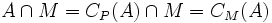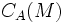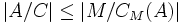# Thompson's lemma on product with centralizer of commutator with abelian subgroup of maximum order

## History

This lemma explicitly appears in Gorenstein's text on Finite Groups (see the reference below). It was part of Thompson's proof of his replacement theorem -- however, Thompson did not state it as a separate lemma.

## Statement

Suppose$P$ is a group of prime power order. Let$\mathcal{A}(P)$ denote the set of abelian subgroups of maximum order in$P$. Suppose$A \in \mathcal{A}(P)$. Suppose$x \in P$ is such that the commutator$[x,A]$ is an abelian subgroup$M$ of$P$. Let$C = C_A(M)$. Then,$MC \in \mathcal{A}(P)$.

The operation sending$A$ to the new subgroup$MC$ is termed (on this wiki) the Thompson replacement operation.

## Facts used

1. Equivalence of definitions of maximal among abelian subgroups: An abelian subgroup of a group that is not contained in any bigger abelian subgroup is a self-centralizing subgroup: it equals its own centralizer.
2. Product formula
3. Formula for commutator of element and product of two elements
4. Witt's identity

## Proof

Given: A group$P$ of prime power order.$\mathcal{A}(P)$ is the set of abelian subgroups of maximum order in$P$. A subgroup$A \in \mathcal{A}(P)$. An element in$x \in P$ such that$M = [x,A]$ is abelian.$C = C_A(M)$.

To prove:$MC \in \mathcal{A}(P)$.

Proof:

1.$MC$ is abelian: By assumption,$M$ is abelian. Since$C = C_A(M) \le A$,$C$ is also abelian. Further, every element of$M$ commutes with every element of$C$ by definition, so$MC$ is abelian.
2.$C_P(A) = A$: Since$A$ is abelian of maximum order, it is maximal among abelian subgroups: it is not contained in a bigger abelian subgroup. Thus, by fact (1),$C_P(A) = A$.
3.$C \cap M = A \cap M = C_M(A)$: Clearly, since$C \le A$,$C \cap M \le A \cap M$. Conversely, anything in$A \cap M$ is in particular inside$M$, so centralizes$M$, hence is in$A \cap C_P(M) = C$. Thus,$A \cap M \le C \cap M$, and we get$C \cap M = A \cap M$. Finally, since step (2) yields$C_P(A) = A$, we get$A \cap M = C_P(A) \cap M = C_M(A)$.
4.$|MC| = |M||C|/|C_M(A)|$: By fact (2),$|MC| = |M||C|/(|C \cap M|)$. By step (3),$C \cap M = C_M(A)$. Thus, we get$|MC| = |M||C|/|C_M(A)|$.
5. Consider the map$a \mapsto [x,a]$ from$A$ to$M = [x,A]$. Then, if$[x,u]$ and$[x,v]$ are in the same coset of$C_M(A)$, then$[x,u^{-1}v] \in C_M(A)$: Suppose$[x,u]$ and$[x,v]$ are in the same coset of$C_M(A)$ in$M$. Then, by fact (3),$[x,v][x,u]^{-1} = c_u([x,u^{-1}v])$. Since the left side is in$C_M(A)$, so is the right side. By definition of$C_M(A)$, we get that$[x,u^{-1}v] \in C_M(A)$.
6. If$a \in A$ and$[x,a] \in C_M(A)$, then$a \in C_A(M)$: If$[x,a] \in C_M(A)$, then$[[x,a],b]$ is the identity for all$b \in A$. By fact (4), and the abelianness of$A$, we obtain that$[[x,c],a]$ is the identity for all$c \in A$. In particular,$a$ commutes with$M$, so$a \in C_A(M)$.
7. The map$a \mapsto [x,a]$ from$A$ to$M$ has the property that if$[x,u]$ and$[x,v]$ are in the same coset of$C_M(A)$, then$u,v$ are in the same coset of$C_A(M)$. Thus, it is an injective map from$A/C_A(M)$ to$M/C_M(A)$: This follows from the previous two steps.
8.$|A/C| \le |M/C_M(A)|$, hence$|A|/|C| \le |M|/|C_M(A)|$: This follows from the previous step.
9. From steps (4) and (6), we get$|MC| \ge |A|$.